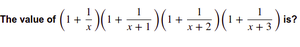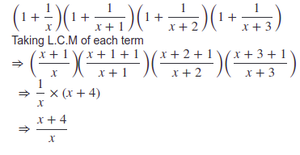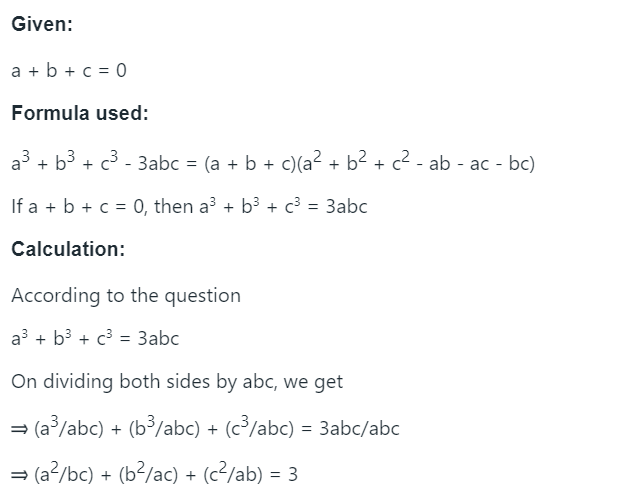# Algebra

• Last Updated : 27 Sep, 2023

 Question 1

Given two numbers x and y such that

1. x3 + y3 = 9

2. x + y = 3

Find the value of x4+y42/371317

Question 1-Explanation:
```x3+y3 = (x + y) × (x2 − xy + y2)

Putting given values of x3+y3 and (x + y)
9 = 3 × ((x+y)2 − 3xy)
= 3 × (9 − 3xy)
= 27 − 9xy

9xy = 18
xy = 2

x4 + y4 = (x2 + y2)2 - 2x2y2
= (x2 + y2)2 - 2*4
[Putting value of xy]
= ((x + y)2 - 2xy)2 - 2*4
[Putting values of (x+y) and xy]
= (9 - 4)2 - 2*4
= 17```
 Question 2

The value of x+1/2x is given as 2, then find the value of 8x3+1/x340/320/72840

Question 2-Explanation:

x3 + 1/8x3 + 3.x.1/2x(x + 1/2x) = (x+1/2x)3

x3 + 1/8x3 + 3 = 23

x3 + 1/8x3 = 5

8x3 + 1/x3 = 5.8 = 40

 Question 3

If x takes only real numbers. For what value of x, the value of the expression: 4−6x−x2 will be maximum?-4-303

Question 3-Explanation:

Differentiate and equate to 0 6+2x =0 x=−3

 Question 4

For what value of x, the following equation: 5√x +12√x =13√x will be true?-203None of the above

Question 4-Explanation:

say x=1
17!=13

for, x=2 and 3 also not possible
x=4
52+122=132
169=169

 Question 5

Solve for x in the equation: log(2x - 1) + log(3) = log(x + 5)x = -1/3x = 1/3x = 5/3x = 8/5

Question 5-Explanation:

Using the property of logarithms that states log(a) + log(b) = log(ab), we can simplify the equation as log(3(2x - 1)) = log(x + 5). Therefore, we have 6x - 3 = x + 5. Simplifying, we get 5x = 8, which gives us x = 8/5. Hence, the answer is D) x = 8/5.

 Question 6

If the value of b2 + 1b2 = 1, then find the value of b3 + 1b3-1/12-2-4None of the above

Question 6-Explanation:
`E = (b + 1⁄b)(b2 - 1 + 1⁄b2)`
 Question 71 + 1/(x+4)x+41/x(x+4)/ x

Question 7-Explanation:Question 8

If a+b+c = 0, then the value of (a^2/bc) + (b^2/ac) + (c^2/ab) will be?3210

Question 8-Explanation:Question 9
Following instructions are to be used throughout the quiz:

Each of the questions given below consists of a statement and/or a question and two statements numbered I and II given below it. You have to decide whether the data provided in the statement(s) is/are sufficient to answer the given question.
(a) if the data in Statement I alone is sufficient to answer the question, while the data in Statement II alone is not sufficient to answer the question.
(b) if the data in Statement II alone is sufficient to answer the question, while the data in Statement I alone is not sufficient to answer the question.
(c) if the data in each Statement I and Statement II alone is sufficient to answer the question.
(d) if the data even in both Statements I and II together are not sufficient to answer the question.
(e) if the data in both Statements I and II together are necessary to answer the question.

If x,y are integers, then (x2 + y2)1/2 is an integer?
I) x2 + y2 is an integer
II) x2 - 3y2 = 0ABCDE

Question 9-Explanation:
Statement 1: x2 + y2 is an integer Since x and y are integer x2+y2 can be any real number which may not be a perfect square so statement 1 alone cant prove whether (x2+y2)(½) is an integer or not. Statement 2: x2- 3y2 = 0 =>x2+y2-4y2=0 =>x2+y2=4y2 => (x2+y2)(½)= 2y Which is an integer because y is an integer.
 Question 10
4/15 of 5/7 of a number is greater than 4/9 of 2/5 of the same number by 8. What is half of that number?63063315105

Question 10-Explanation:
Let the number be x. Then, 4/15 of 5x/7 = 4/9 of 2x/5 + 8 4/15 * 5x/7 = 4/9 * 2x/5 + 8 4x/21 = 8x/45 + 8 x/21 = 2x/45 + 2 x/21 = (2x + 90)/45 45x = 21(2x + 90) 45x = 42x + 90*21 3x = 90*21 x = 30*21 = 630. Half of the number will be x/2 x/2 = 630/2 = 315.
There are 20 questions to complete.